# Turan, Paul

## Turán's problem for hypergraphs ★★

Author(s): Turan

Conjecture   Every simple-uniform hypergraph on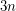vertices which contains no complete-uniform hypergraph on four vertices has at most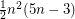hyperedges.
Conjecture   Every simple-uniform hypergraph on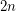vertices which contains no complete-uniform hypergraph on five vertices has at mosthyperedges.

Keywords:

## The Erdos-Turan conjecture on additive bases ★★★★

Author(s): Erdos; Turan

Let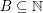. The representation function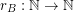for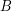is given by the rule. We callan additive basis ifis never.

Conjecture   Ifis an additive basis, thenis unbounded.

The crossing numberofis the minimum number of crossings in all drawings ofin the plane.
Conjecture